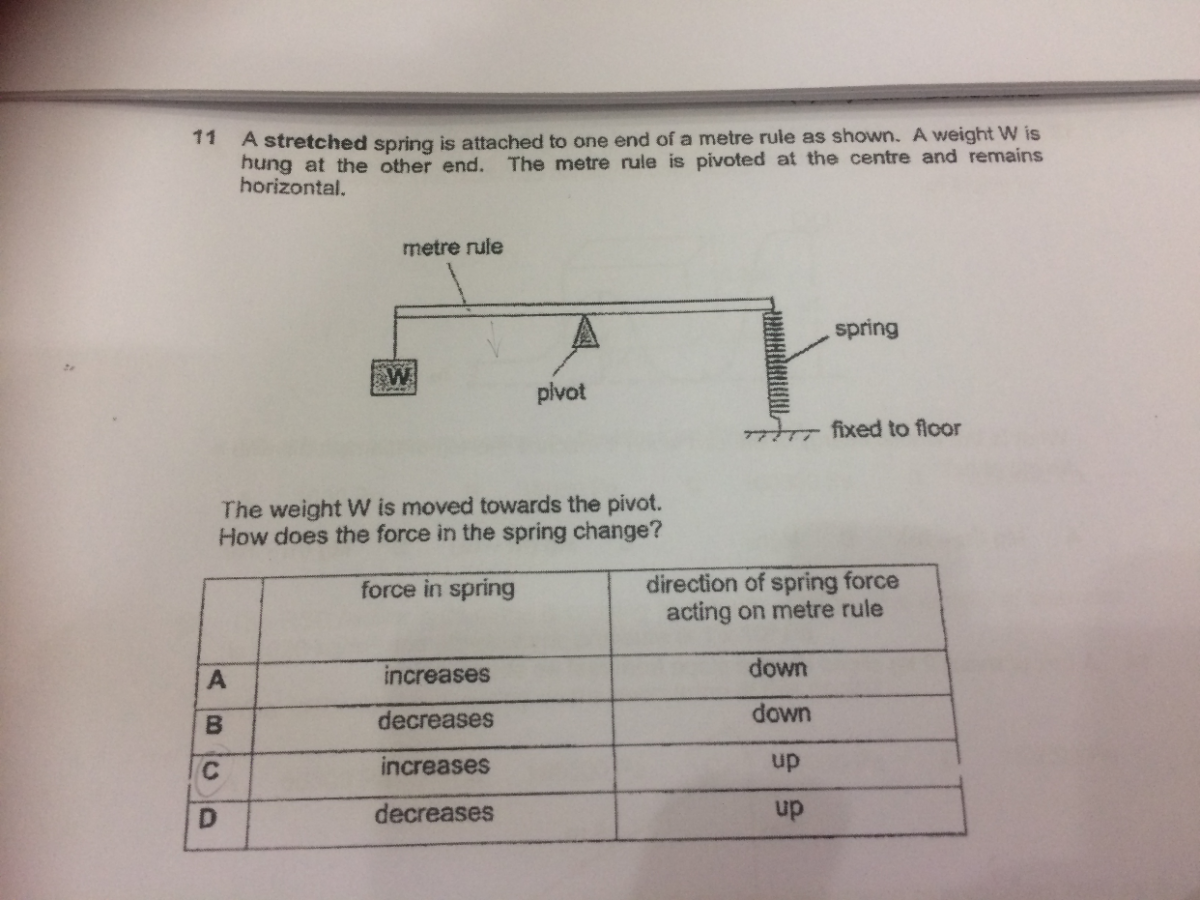# QuestionHi

Can someone explain why answer is C?

1 Answer

# Answer

I think answer is wrong, below example by calculation. Anyone, pls verify. Thanks.

In equilibrium:                Anti-clockwise torque by W = Clockwise torque by Spring

at mid point:                                  Fw (N) x 0.5 (m)       = Fs (N) x 0.5 (m)

as W moves to pivot, e.g.:      same Fw (N)  x 0.2 (m) = Fs’ (N) x 0.5 (m)   ->lower torque on both sides

So Fs’ should be lower (Decreased downward force of spring acting on the ruler) – Answer (B)

1 Reply 2 Likes ✔Accepted Answer

Hi SAHMom

My answer is B too. Thank you so much for your clarification. Thanks again!

0 Replies 1 Like